# Distributinator: Scalable Big-Data Analytics

We design novel distributed algorithms for data-intensive computations in the cloud, asking questions like these:

•  How do we effectively and efficiently use many machines in a cluster or in a cloud to solve a big-data-analysis challenge?

•  What is the best way to partition a dataset so that running time or monetary cost of the distributed computation is minimized?

•  How do we abstract a complex distributed computation so that we can learn a mathematical model of how running time depends on parameters affecting data partitioning?

We have focused on SQL queries involving joins, but we are working on extensions to other applications and problem types, including graphs and data lakes.

## Problem Overview

While we have explored a variety of data-intensive computation problems, the discussion here will be limited to selected highlights for distributed computation of a variety of joins. The join operator is arguably the most important building block of any non-trivial SQL query. The join computation pattern also appears in many other contexts, including graph-pattern search, exploratory analysis, and correlation analysis. For instance, finding a path of length 2 in a graph is equivalent to selecting all pairs of edges with matching endpoints (equality condition on "to" node of first edge and "from" node of second edge) over a join of the edge set with itself. Note that we are not arguing for or against using relational DBMS for graph analytics. We simply are interested in the computational pattern and then take a fresh look at its distributed implementation.

So, let's dive right in and explore a specific type of (expensive) join: the Cartesian product aka cross product. Given two input tables S and T, it returns all pairs (s,t) of tuples s from S and t from T. Assume we have two worker machines w1 and w2. How should we partition S and T?

Let's cut S in half into S1 and S2, and assign S1 to w1 and S2 to w2. What happens to T? Since all S-tuples have to be paired up with all T-tuples, we must copy T and send it to both workers! The figure below illustrates this idea for 4 workers. (The join matrix represents the space of all possible pairs of S- and T-tuples.)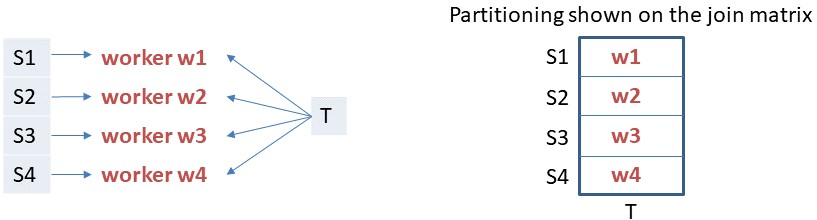What if we have 100 workers w1 to w100? We can now cut S into 100 equal pieces and distribute them evenly over the 100 workers. Unfortunately, we now need 100 copies of T to be able to compute the full Cartesian product. This approach is the classic partition-broadcast. (S is partitioned, while T has to be broadcast.)

Can we do better? What if we cut S into 10 equal-sized pieces S1,..., S10 and do the same for T? How do we assign these S and T partitions to the 100 workers? Consider the following: The first 10 workers all receive S1, but each gets a different partition of T. The next 10 workers all receive S2 and each a different partition of T, and so on. Then each worker computes its local Cartesian product between the S and T partition it received. This is illustrated below for a 4-worker scenario.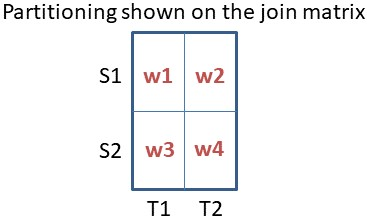Will this correctly compute the Cartesian product of S and T? This is actually easy to prove. Consider any output tuple (s,t). Clearly, s has to be in some partition of S, say Si, and t in some partition Tj. The way we assigned partitions guarantees that there is exactly one worker that received Si and Tj. This means that this worker will output (s,t) and no other worker can produce it. (Nine other workers received Si, but none of them received Tj; and vice versa.)

Is this really better than partition-broadcast? Partition-broadcast works with 1 copy of S and 100 copies of T, assigning 0.01|S|+|T| input data to each worker. (We use |X| to denote the size of set X. For simplicity we do not distinguish between size and cardinality of a set here.) The 10-by-10 scheme assigns 0.1|S|+0.1|T| per worker. The table below compares per-worker input for different scenarios of the size of S vs T. Interestingly, no scheme dominates the other. Depending on the relative size of S vs T, one or the other may assign up to an order of magnitude less input per worker!

Size of S Size of T Worker input (partition-broadcast) Worker input (10-by-10) Worker-input ratio
n n 1.01n 0.2n 5.05
n 1000n 1000.01n 100.1n 9.99
1000n n 11n 100.1n 0.11

## Result Highlights

We discuss selected highlights; for details check out our papers below. There are many remaining challenges in this exciting research area. And we are looking for PhD students and postdocs to explore them!

Near-optimal distributed theta-joins: The theta-join is defined as any subset of the Cartesian product as identified by a Boolean function that returns true or false for a given pair (s,t) of tuples from S and T, respectively. Starting with the above observations for the Cartesian product, we developed a variety of strong theoretical and empirical results:

• We proposed the 1-Bucket algorithm (called 1-Bucket-Theta in the paper; discussed more concisely and intuitively here). It does not need any cost model: only the size of S and T. (Hence the name: its required statistics are equivalent to the information contained in a frequency-distribution histogram that has a single bucket or bin, i.e., only reports the total count.) For the Cartesian product, 1-Bucket is provably near-optimal in terms of the amount of input assigned to and output produced by each worker. Somewhat surprisingly, the larger the number of workers, the stronger the guarantee becomes, but it is always within a small constant factor of the lower bound!

• We show that 1-Bucket is also near-optimal for any output-cost dominated join, no matter the type of theta-join Boolean function.

• For joins where output-related costs do not dominate, e.g., typical equi-joins or selective inequality- and band-joins, we propose the M-Bucket family of algorithms. They work with more fine-grained frequency-distribution statistics that correspond to a histogram with M>1 buckets. While they do not provide non-trivial theoretical guarantees, they worked very well on a variety of problems.

Near-optimal distributed equi-joins: The equi-join is arguably the most common type of theta-join. In relational DBMS it is frequently used to join tables based on foreign keys. In graph DBMS it appears in the context of pattern search. It had received a lot of attention in the research community, but we still were able to make exciting new contributions: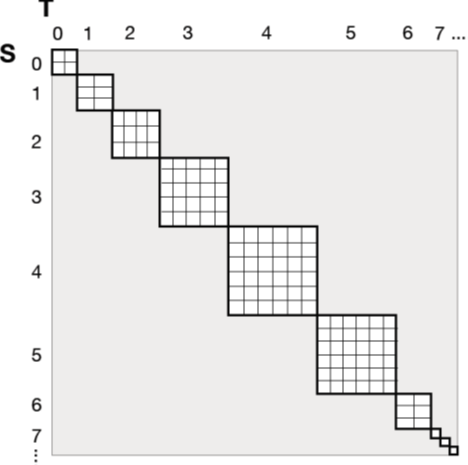• We proposed ExpVar, illustrated above, the best-performing distributed equi-join algorithm (as of early 2020), which can handle even extremely skewed input data. It generalizes the classic hash-partitioning-based approach by considering clever splitting of large partitions.

• Starting with a mathematical model for load assignment, we prove that the resulting join-optimization problem is monotonic and submodular under fairly mild assumptions. This led to a simple greedy heuristic with small-constant-factor approximation guarantees. For practical purposes, such guarantees are much more desirable than even "asymptotic optimality," where the constant factors could be arbitrarily large.

Near-optimal band-joins: Band-joins generalize equi-join and Cartesian product, but the 1-Bucket algorithm is suboptimal for band-joins with relatively small output.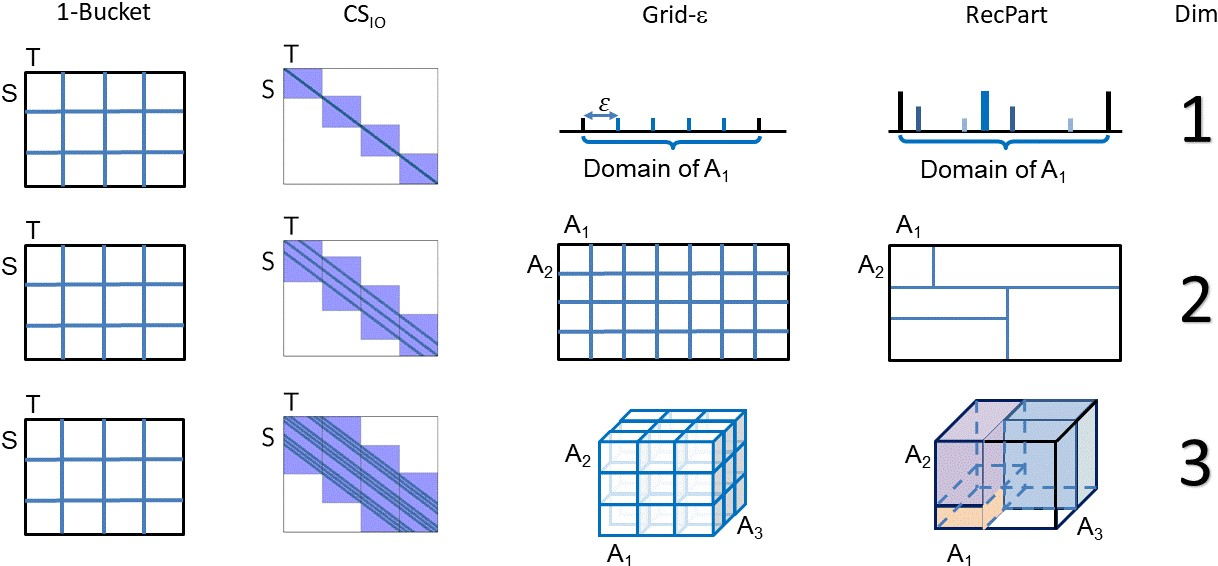Different partitioning strategies for band-joins are shown in the figure above. 1-Bucket and CSIO partition the join matrix where rows correspond to S-tuples and columns to T-tuples. Grid and RecPart partition the space defined by the join attributes.

• We proposed the RecPart recursive-partitioning algorithm inspired by decision trees to assign input tuples to workers. The challenge with band-joins is that often input tuples have to be duplicated across partition boundaries. Due to the difficulty of the problem, we were not able to prove theoretical optimality guarantees.

•  However, across a wide variety of applications, queries, and clusters, RecPart was always within 10% of the lower bound in terms of load-per-worker and input duplication caused by the partitioning scheme. None of the previously proposed approaches comes anywhere near as shown in the figure below!

• These near-optimal partitionings are found very quickly, typically within a couple of seconds when running on a single commodity machine, which is a negligible cost compared to the time it takes to compute the actual join result.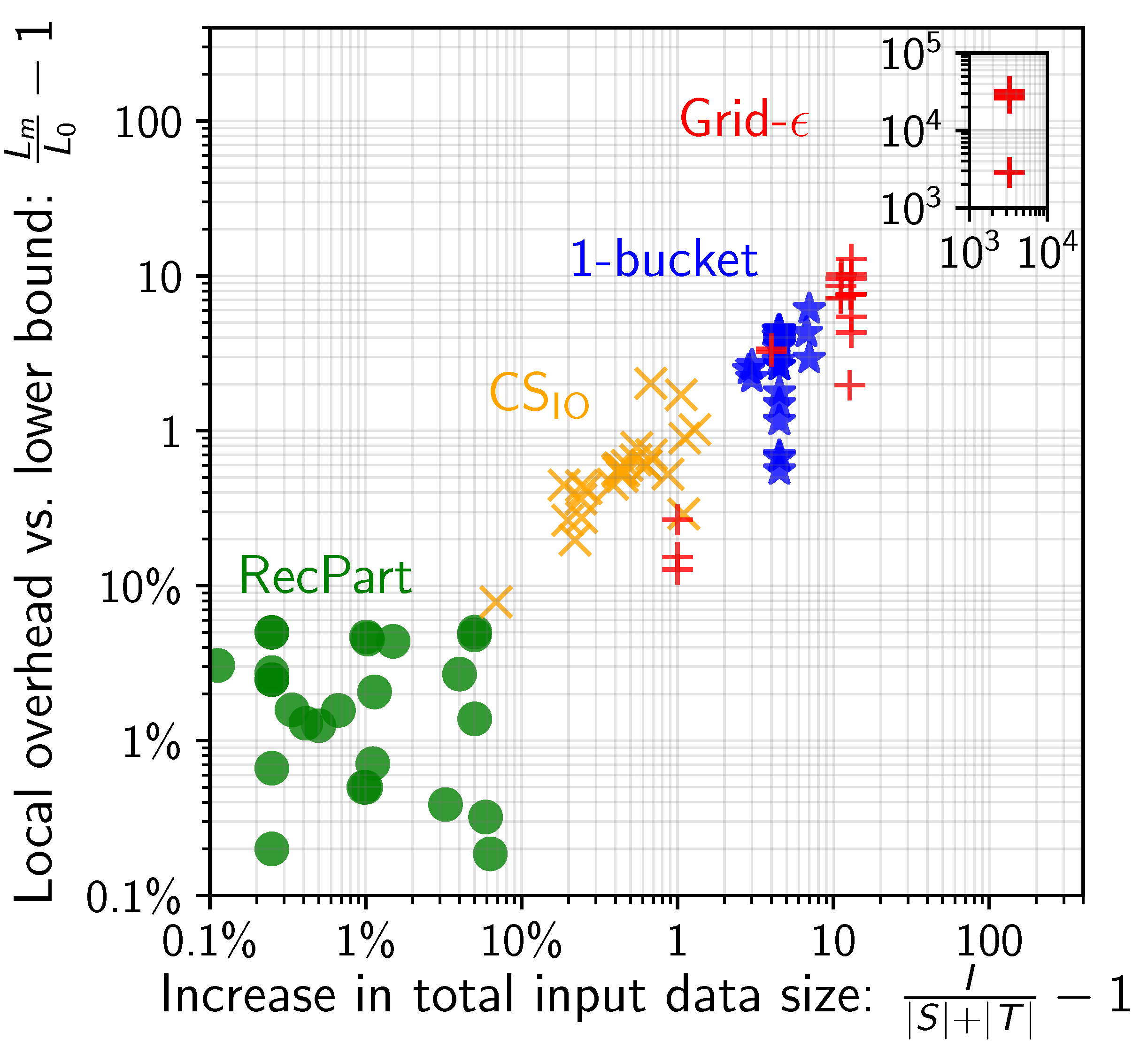Flexible, easy-to-train distributed cost models: Estimating the end-to-end running time for a distributed computation is notoriously difficult. Modern machine-learning approaches have been explored, but they require a lot of training data that in turn requires the execution of large benchmarks for profiling.

• We proposed abstract cost models that carefully navigate the tradeoffs between parametric and non-parametric learning methods. These cost models require comparably little training data, but are still sufficiently accurate for predicting running time of competing data partitionings considered.

• We successfully applied these models to predict running time of distributed sorting, matrix product, equi-joins, and band-joins.

## Publications and Software

Rundong Li, Wolfgang Gatterbauer, Mirek Riedewald. Near-optimal distributed band-joins through recursive partitioning. To appear in Proc. ACM SIGMOD Int. Conf. on Managament of Data, 2020 [preprint]

R. Li, N. Mi, M. Riedewald, Y. Sun, Y. Yao. Abstract Cost Models for Distributed Data-Intensive Computations. Distributed and Parallel Databases, 37(3): 411-439, Springer, 2019 [preprint]

R. Li, M. Riedewald, X. Deng. Submodularity of Distributed Join Computation. In Proc. ACM SIGMOD Int. Conf. on Managament of Data, pages 1237-1252, 2018 [preprint]

R. Li, N. Mi, M. Riedewald, Y. Sun, Y. Yao. A Case for Abstract Cost Models for Distributed Execution of Analytics Operators. In Proc. Int. Conf. on Big Data Analytics and Knowledge Discovery (, pages 149-163, 2017 (invited to special issue of Distributed and Parallel Databases presenting the Best Papers of DaWaK) [paper]

A. Okcan and M. Riedewald. Processing Theta-Joins using MapReduce. In Proc. ACM SIGMOD Int. Conf. on Managament of Data, pages 949-960, 2011

## People

### DATA Lab Team

Xinyan Deng (MS student, first job after graduation: Microsoft)
Wolfgang Gatterbauer